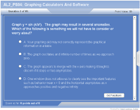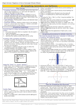How to Learn in 24 Hours?The Rapid Learning Movie

 Need Help? M-F: 9am-5pm(PST): Toll-Free: (877) RAPID-10 US Direct: (714) 692-2900 Int'l: 001-714-692-2900 24/7 Online Technical Support: The Rapid Support Center Secure Online Order:Need Proof? Testimonials by Our Users

 Rapid Learning Courses: MCAT in 24 Hours (2015-16) USMLE in 24 Hours (Boards) Chemistry in 24 Hours Biology in 24 Hours Physics in 24 Hours Mathematics in 24 Hours Psychology in 24 Hours SAT in 24 Hours ACT in 24 Hours AP in 24 Hours CLEP in 24 Hours DAT in 24 Hours (Dental) OAT in 24 Hours (Optometry) PCAT in 24 Hours (Pharmacy) Nursing Entrance Exams Certification in 24 Hours eBook - Survival Kits Audiobooks (MP3)

 Tell-A-Friend: Have friends taking science and math courses too? Tell them about our rapid learning system.Home »  Mathematics »  High School Algebra 2

Graphing Utilities

 Topic Review on "Title": Graphing Aids: A graphing calculator is any handheld device that allows the user to input equations in “y=” form and will output an image of the graph for that input. CASs: Software run on a computer that allows the user to input equations and will provide a graphical image for that input.  Fixed Window: Plotting graphs using a pre-selected range of x and y values. Automatic Window: Plotting graphs using a pre-selected range of x values where the ranges of y values are calculated such that al points fit in the window.

Rapid Study Kit for "Title":
 Flash Movie Flash Game Flash Card Core Concept Tutorial Problem Solving Drill Review Cheat Sheet"Title" Tutorial Summary : Graphing utilities allow for the user to graph equations that would be otherwise difficult to graph with pencil and paper. The user of a graphing calculator can approximate values of mathematical problems that are not exact values. The common miscellaneous ways that using a calculator or other graphing utilities makes calculations easier is mentioned in this tutorial. These mathematical tools can give inaccurate results if they are used improperly. It is good to understand them to prevent from doing them when using graphing utilities.

 Tutorial Features: Specific Tutorial Features: • Examples showing the steps that take to generate plots of equations are discussed. • Subtleties in particular equation plots produced by graphing calculators are mentioned with the use of examples. Series Features: • Concept map showing inter-connections of new concepts in this tutorial and those previously introduced. • Definition slides introduce terms as they are needed. • Visual representation of concepts • Animated examples—worked out step by step • A concise summary is given at the conclusion of the tutorial.

 "Title" Topic List: Graphing Calculators and Software Programs used in Algebra 2Graphing Functions using Graphing Calculators and Software ProgramsSubtleties found when Graphing Special Functions

See all 24 lessons in High School Algebra 2, including concept tutorials, problem drills and cheat sheets:
Teach Yourself High School Algebra 2 Visually in 24 Hours